# Frank Solutions for Class 10 Maths Chapter 5 Linear Inequations

Frank Solutions for Class 10 Maths Chapter 5 Linear Inequations, are available here. A thorough understanding and study of the Frank Solutions will allow students to prepare comprehensively for their examinations and in turn, ace exams effectively. Our topmost experts have answered these questions in such a way that students can understand clearly without any doubts. We suggest students download Frank Solutions for Class 10 Maths Chapter 5 offline as well.

Chapter 5 – Linear Inequations, linear inequality is an inequality which involves a linear function. A linear inequality contains one of the inequalities. This chapter deals with different types of equations and problems based on linear inequalities.

## Download the PDF of Frank Solutions for Class 10 Maths Chapter 5 Linear Inequations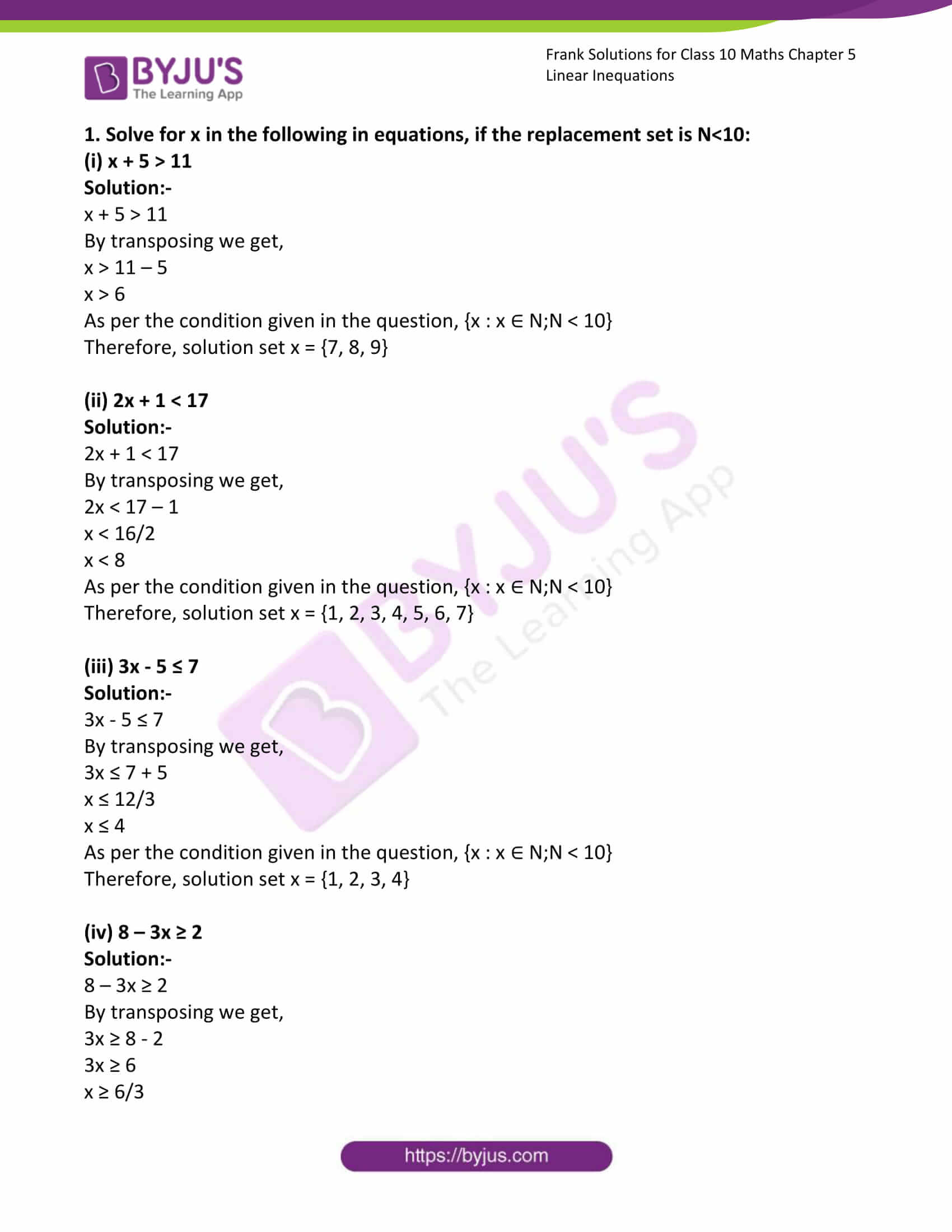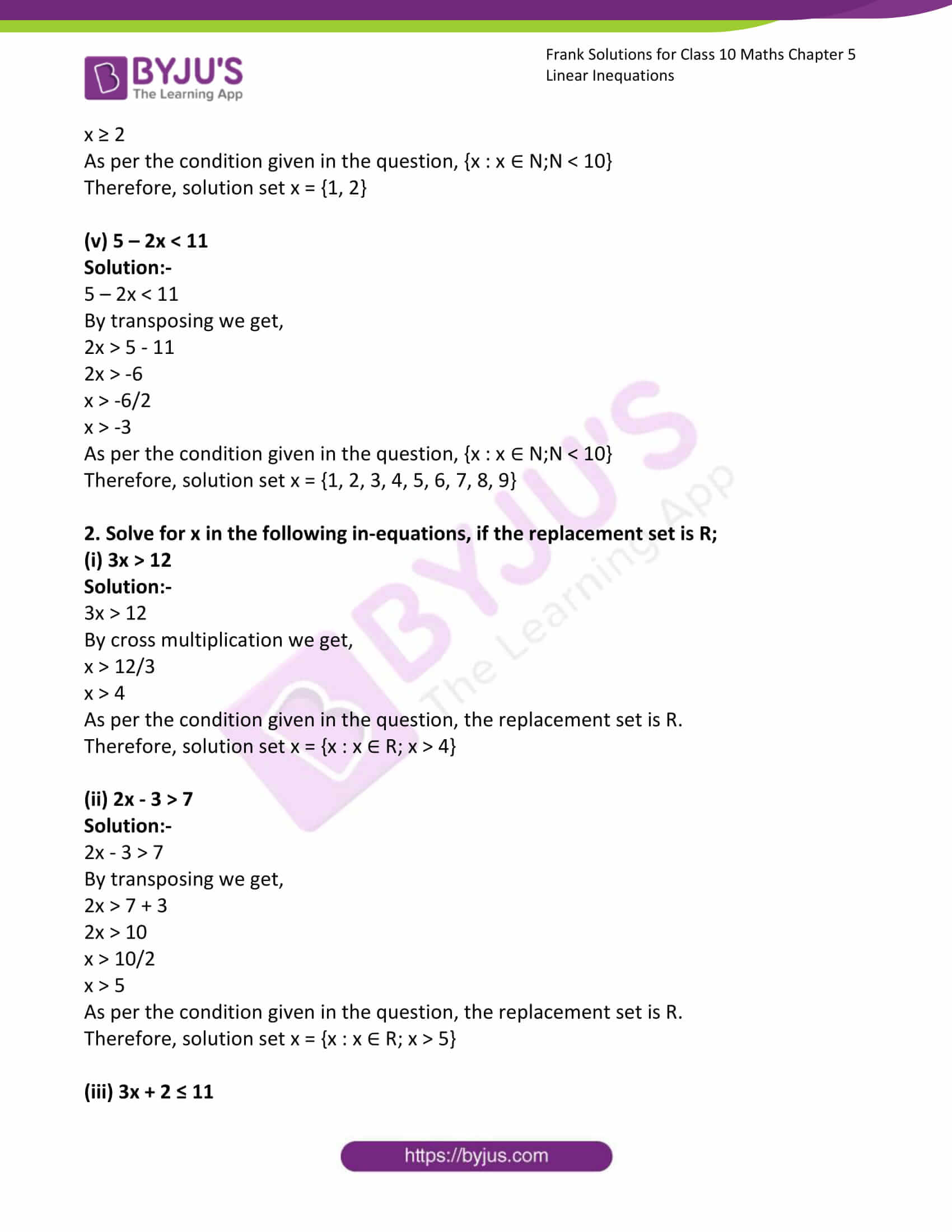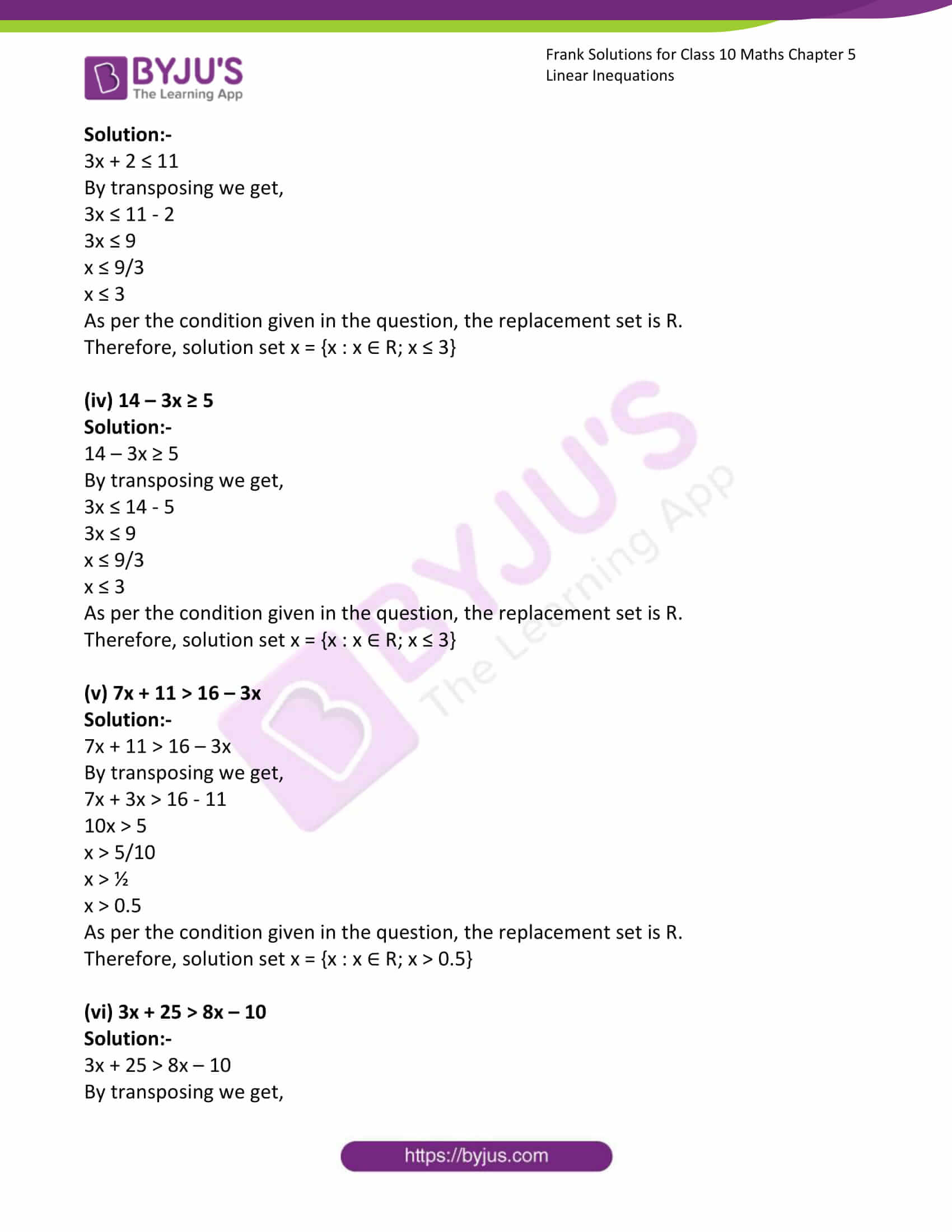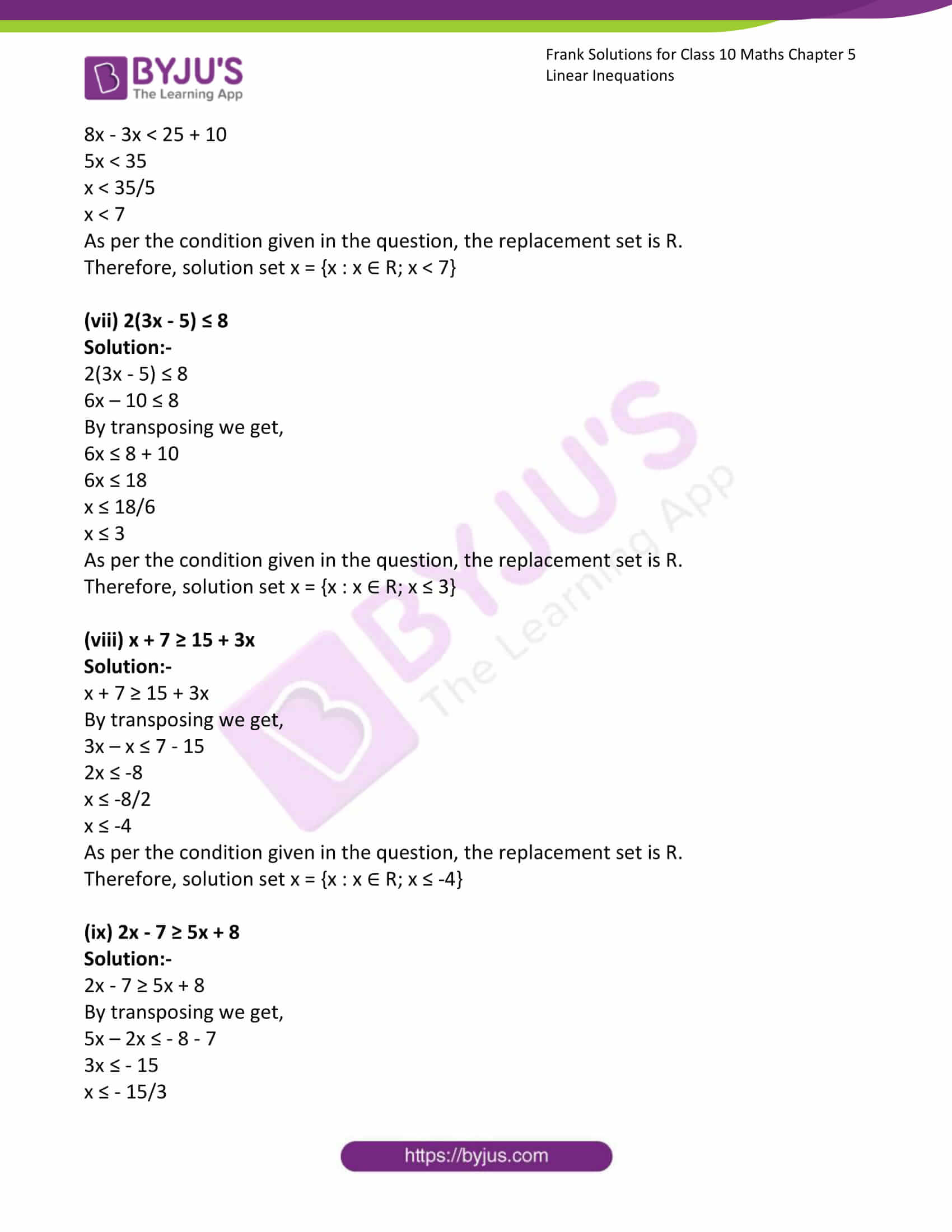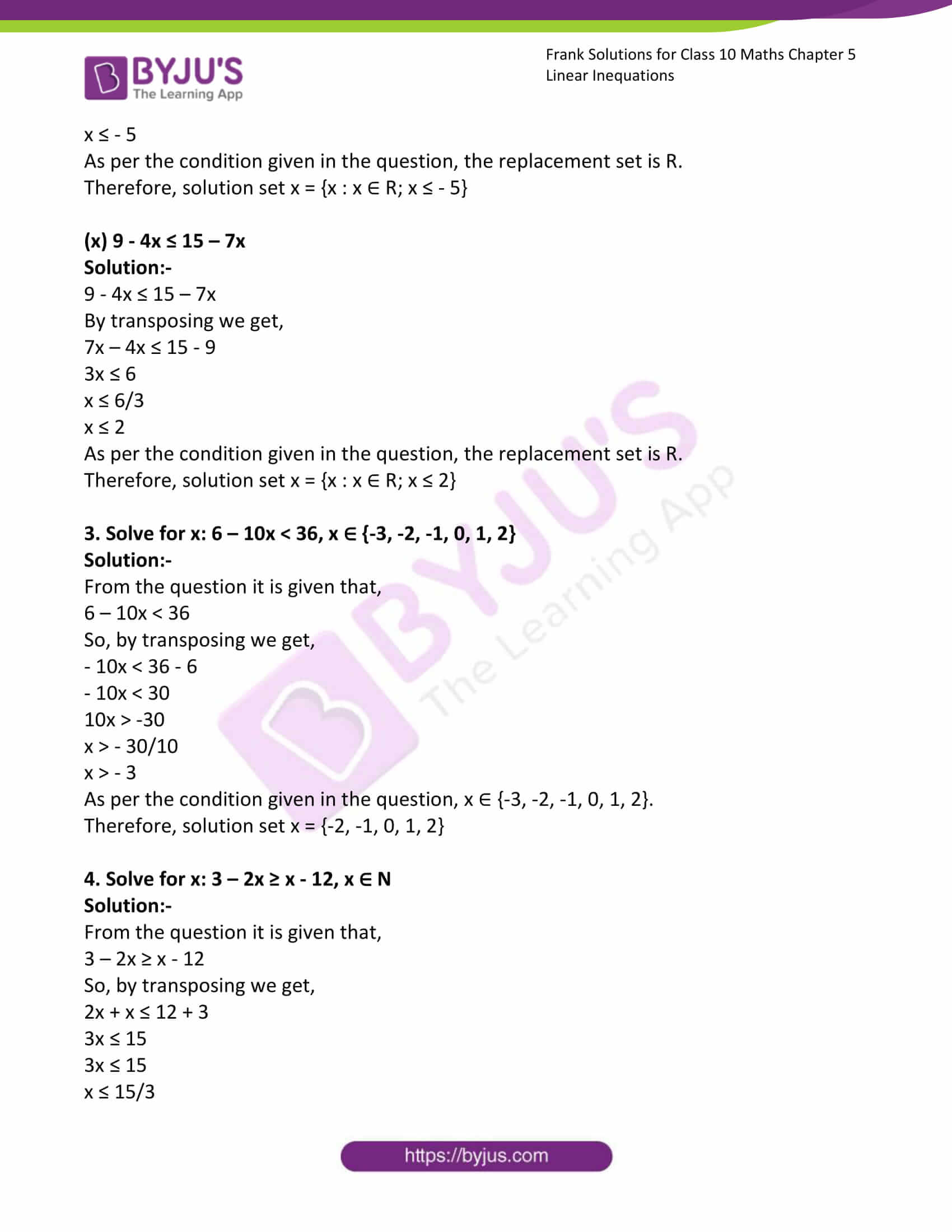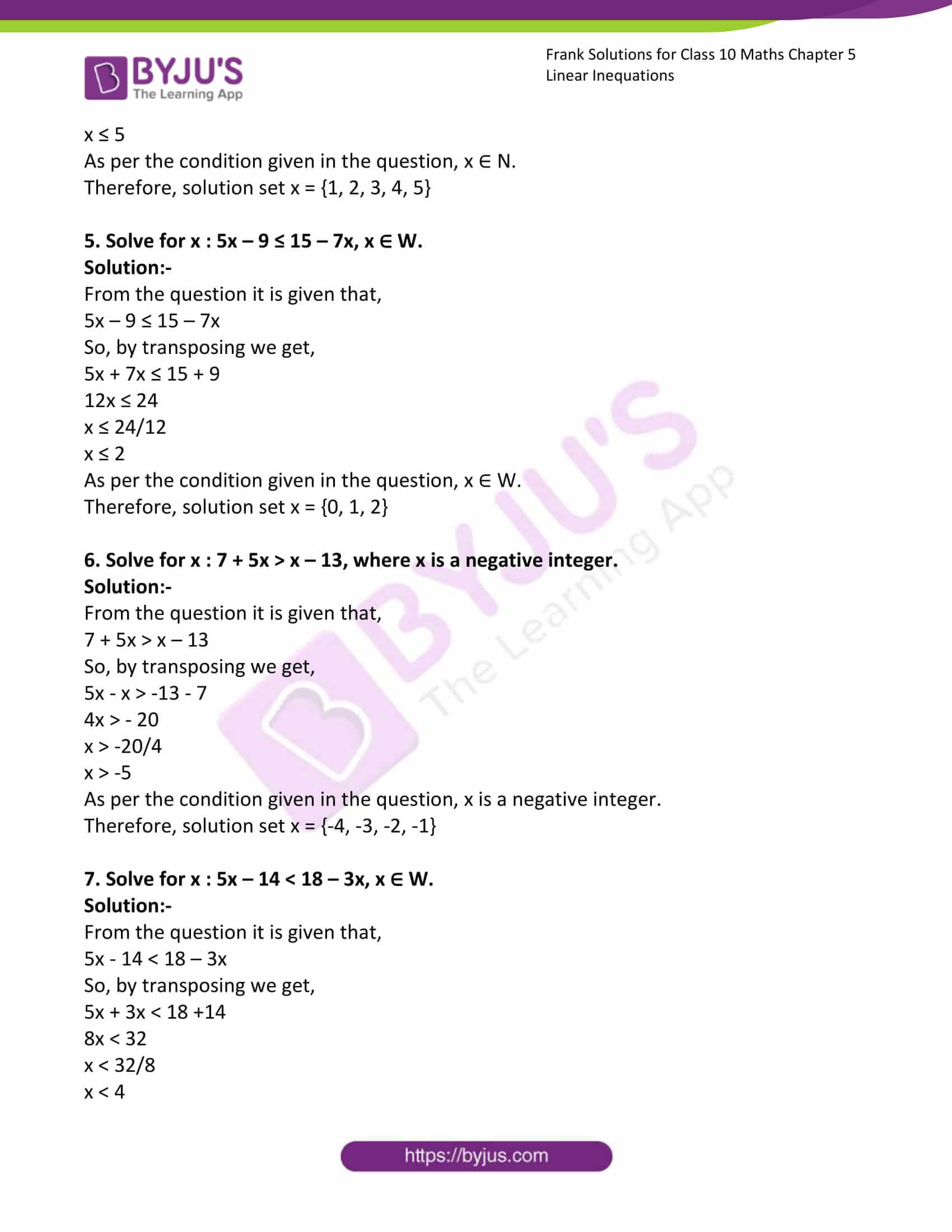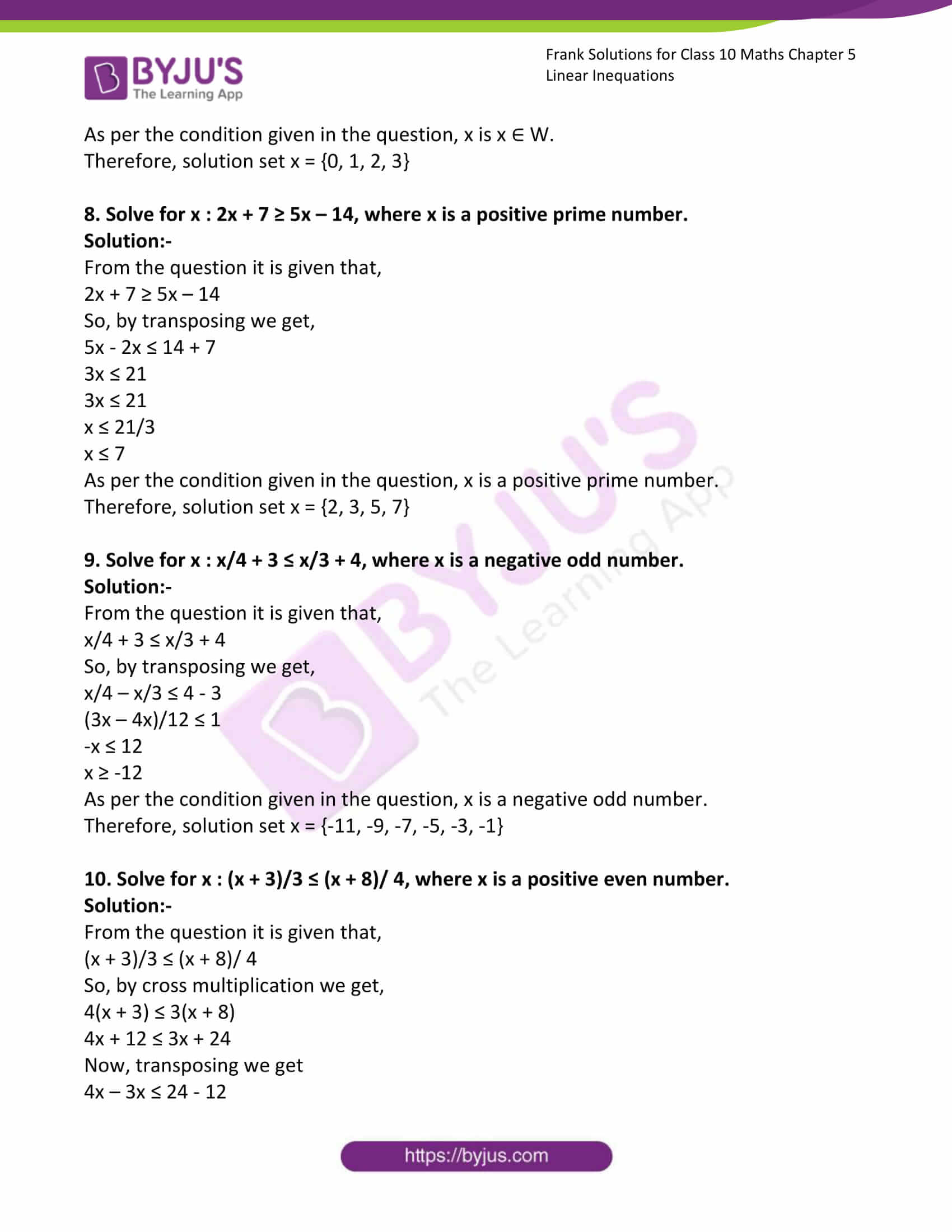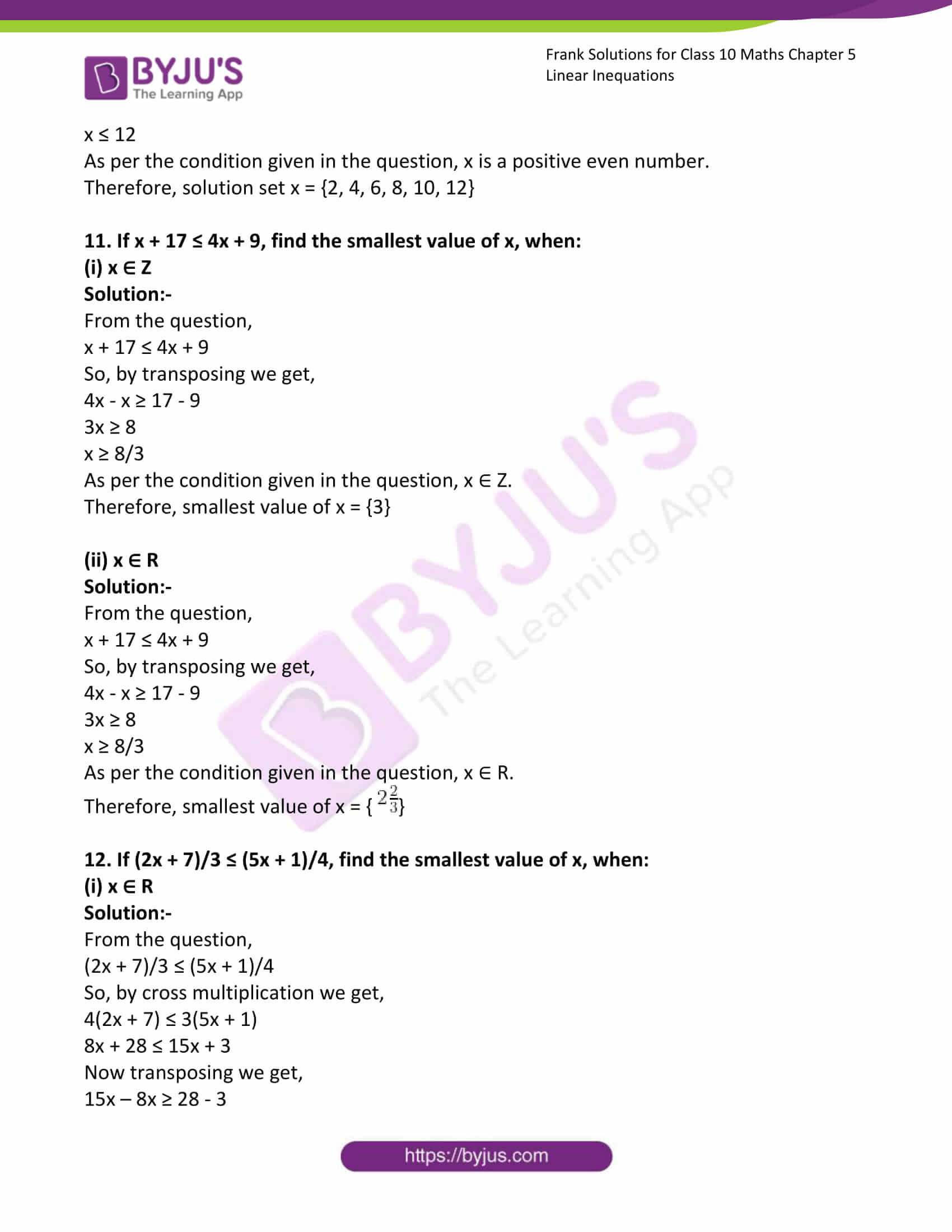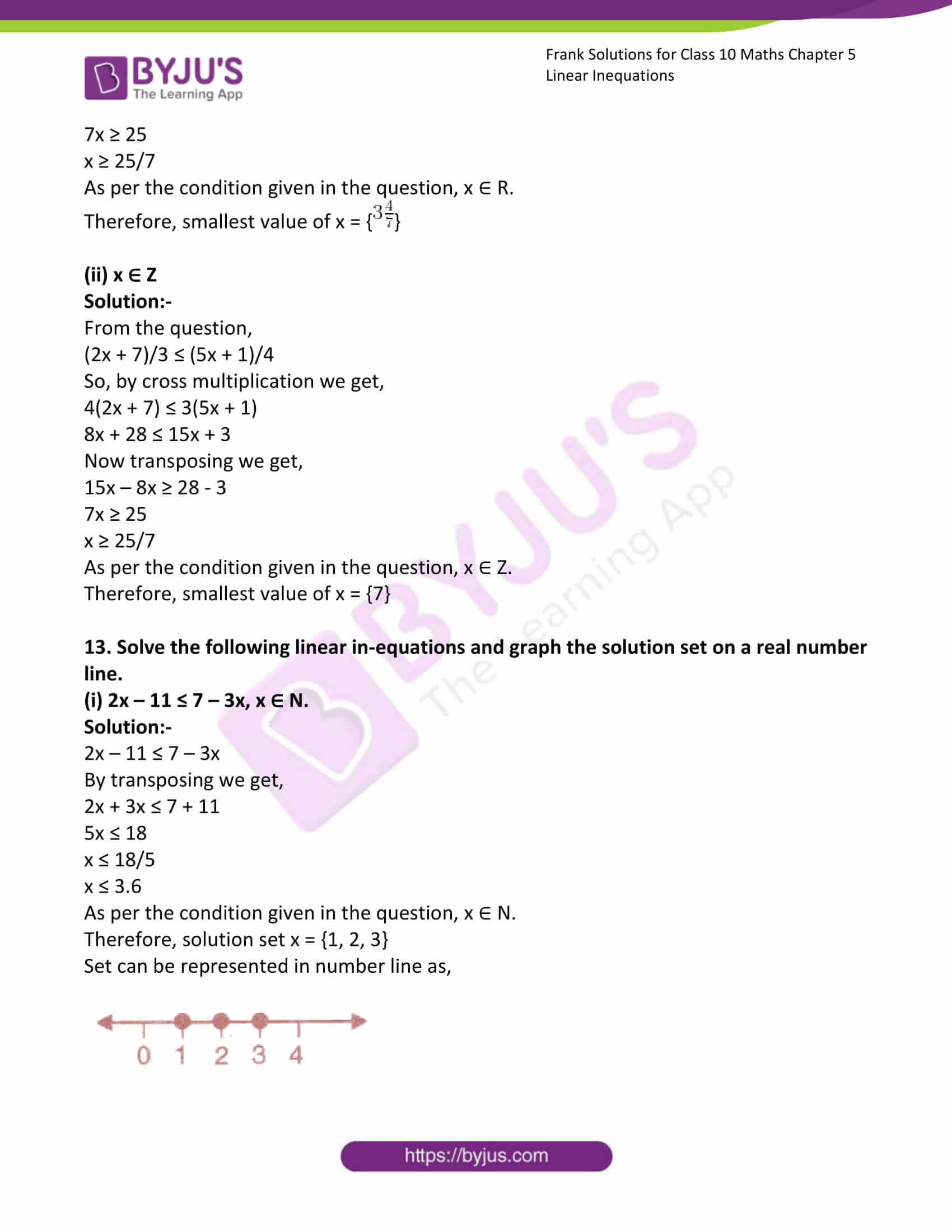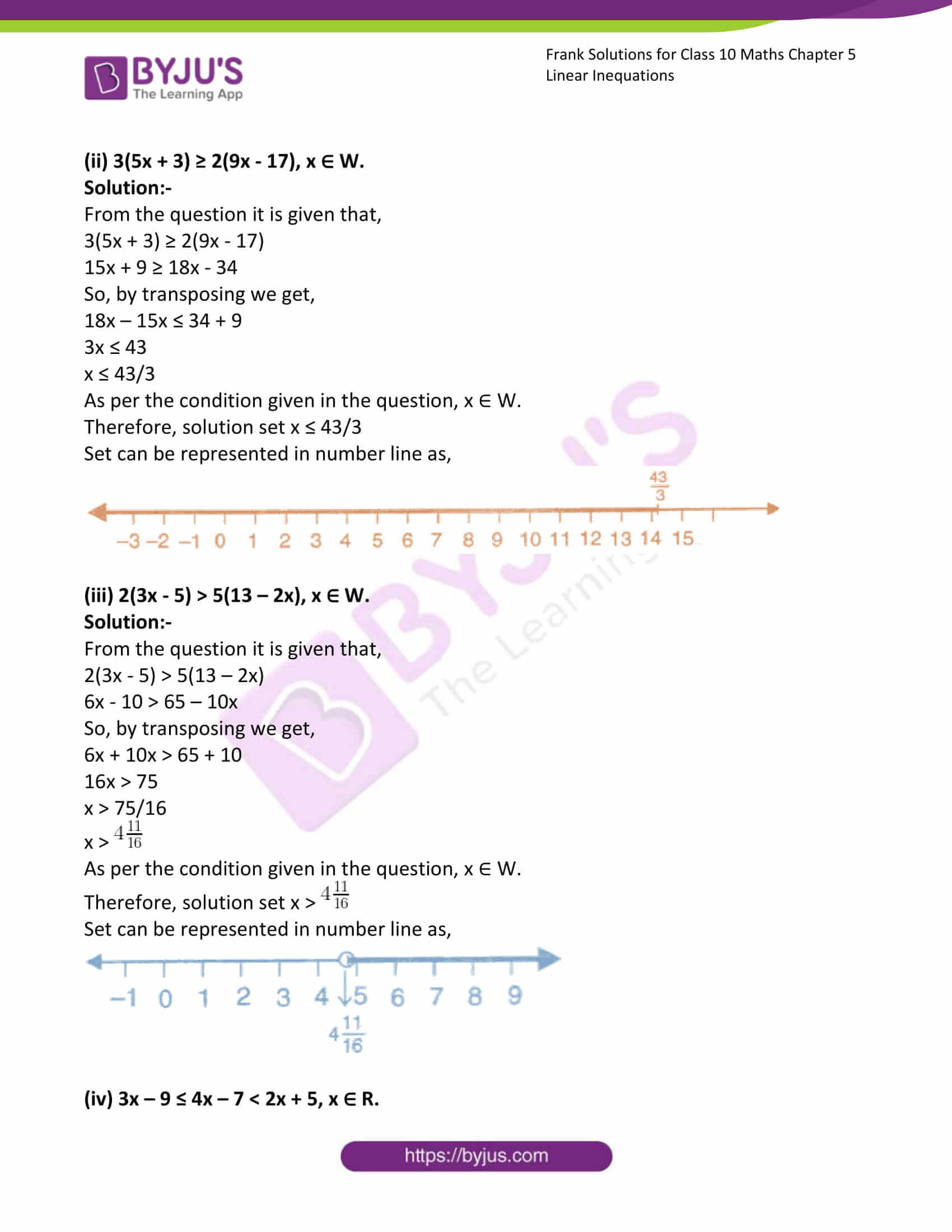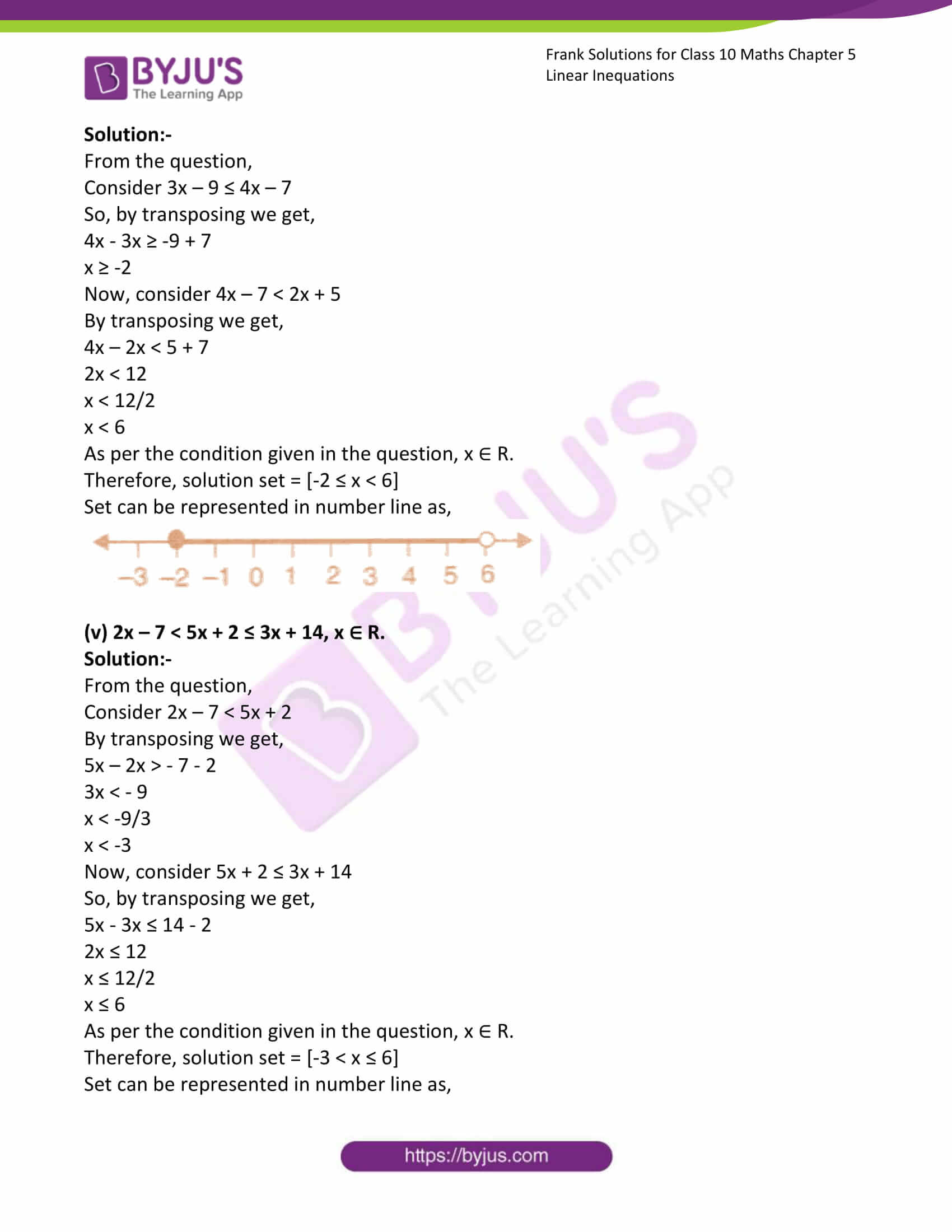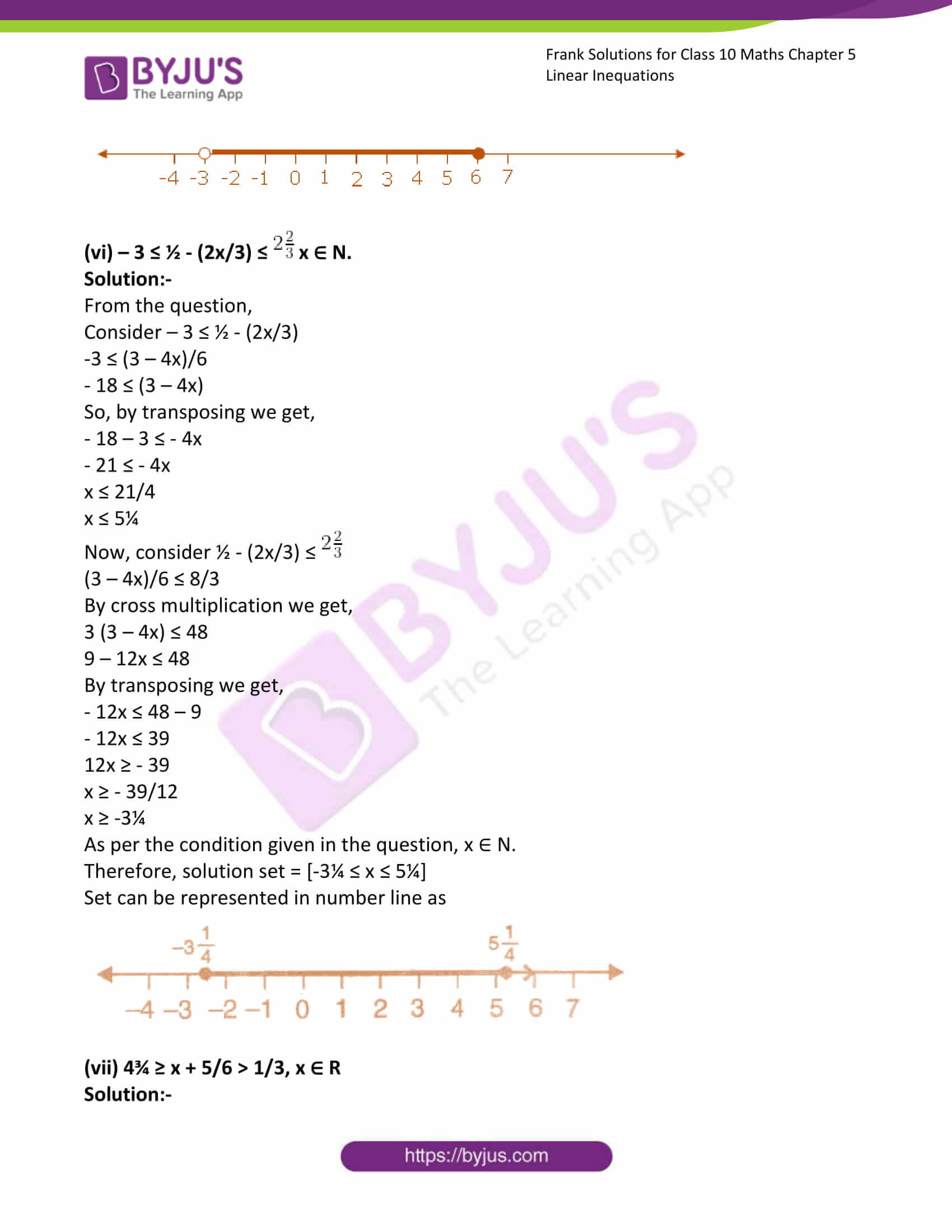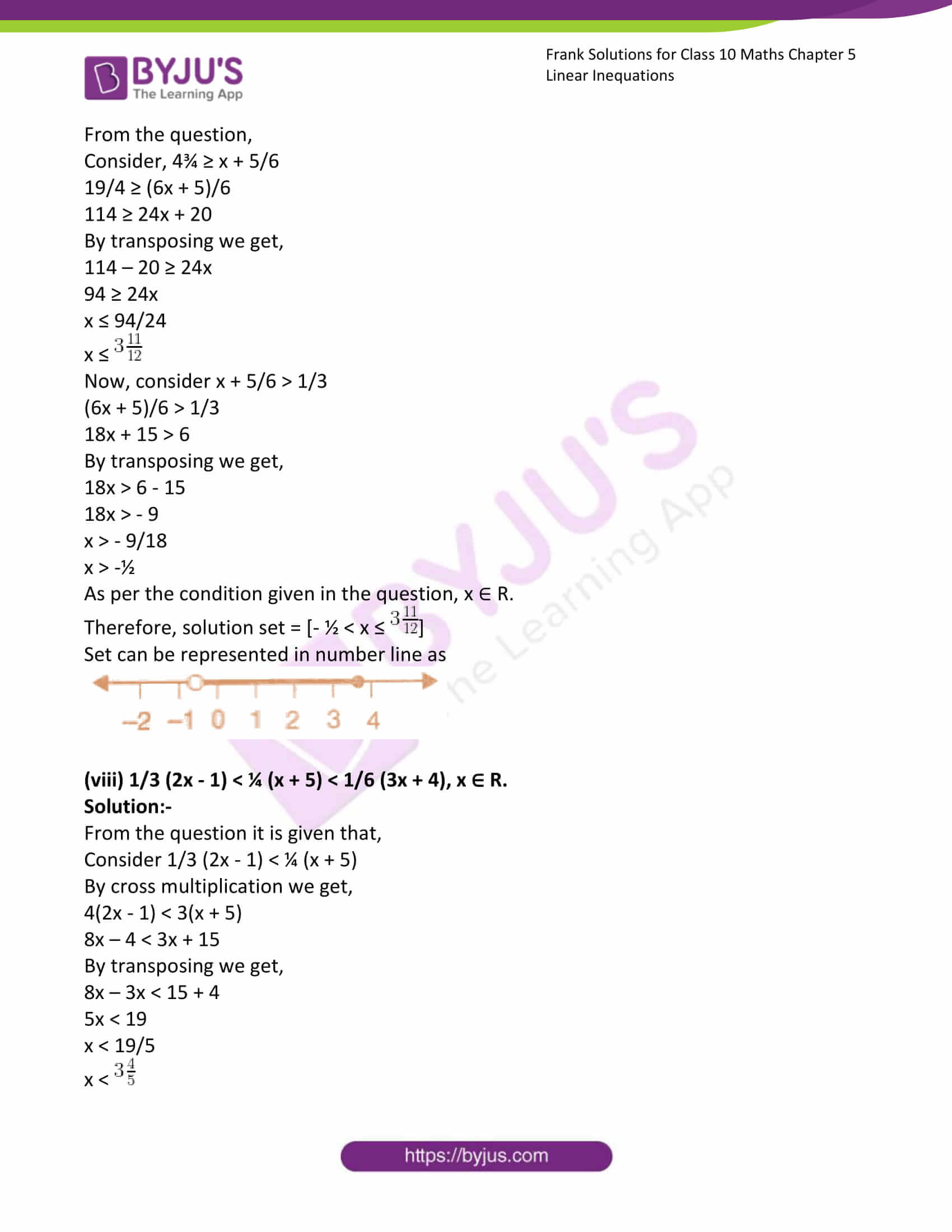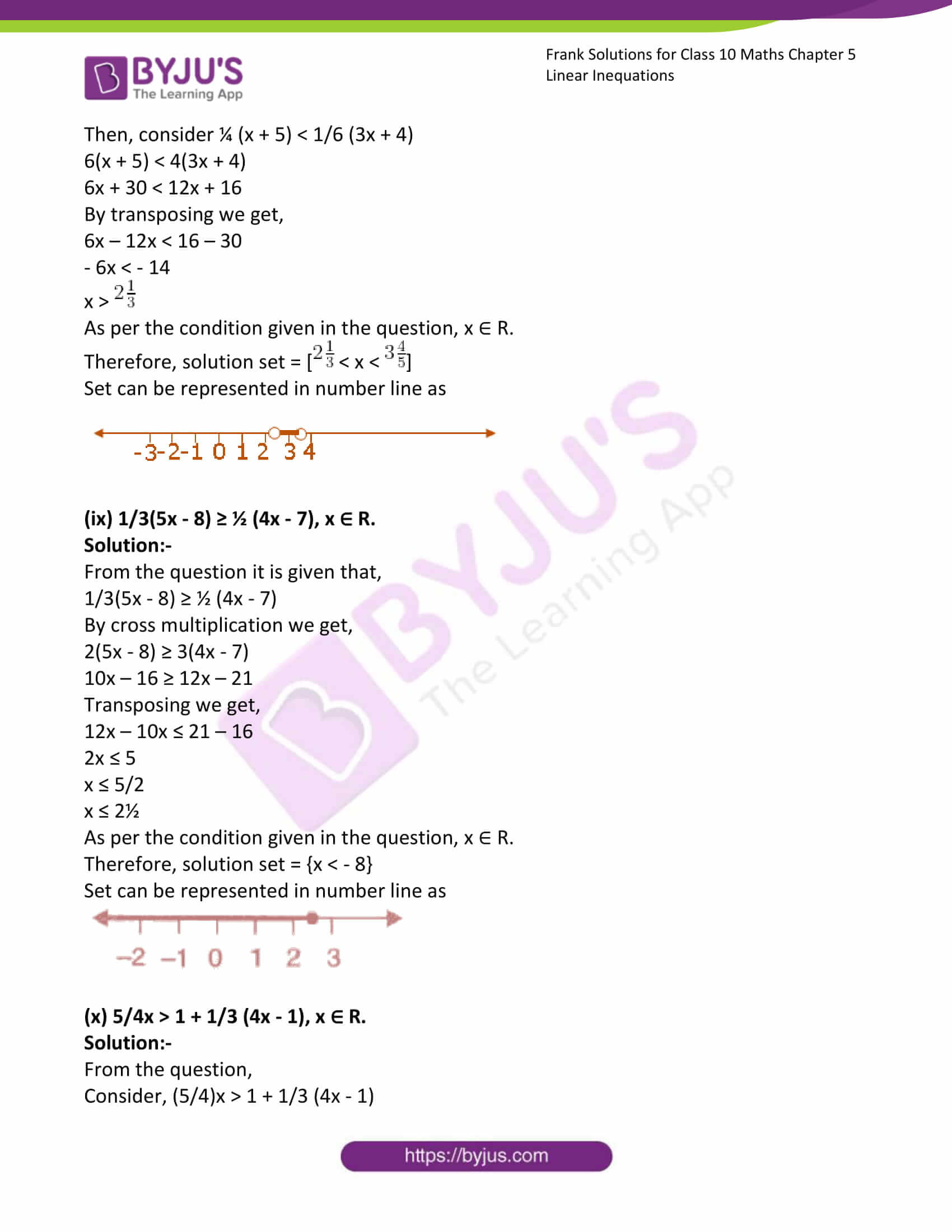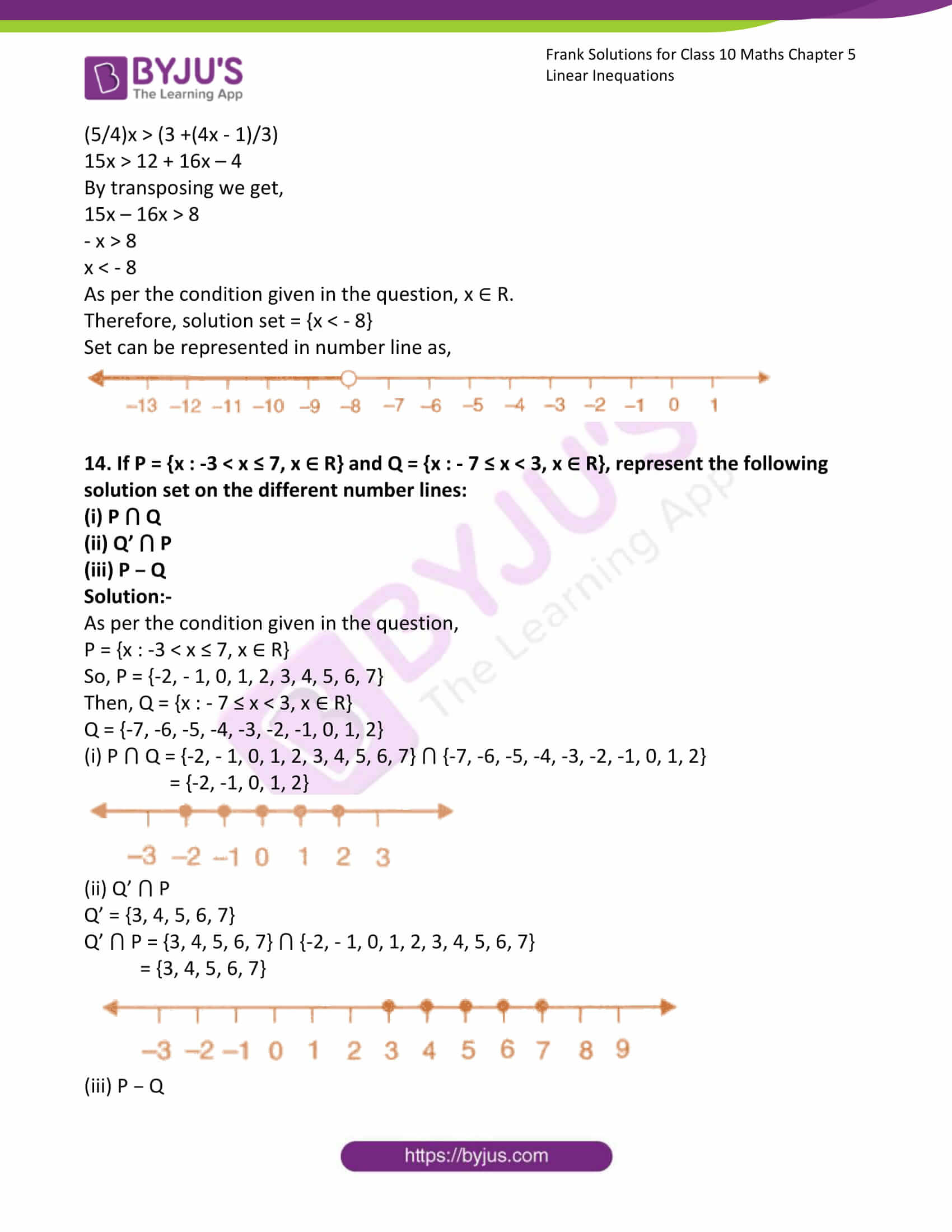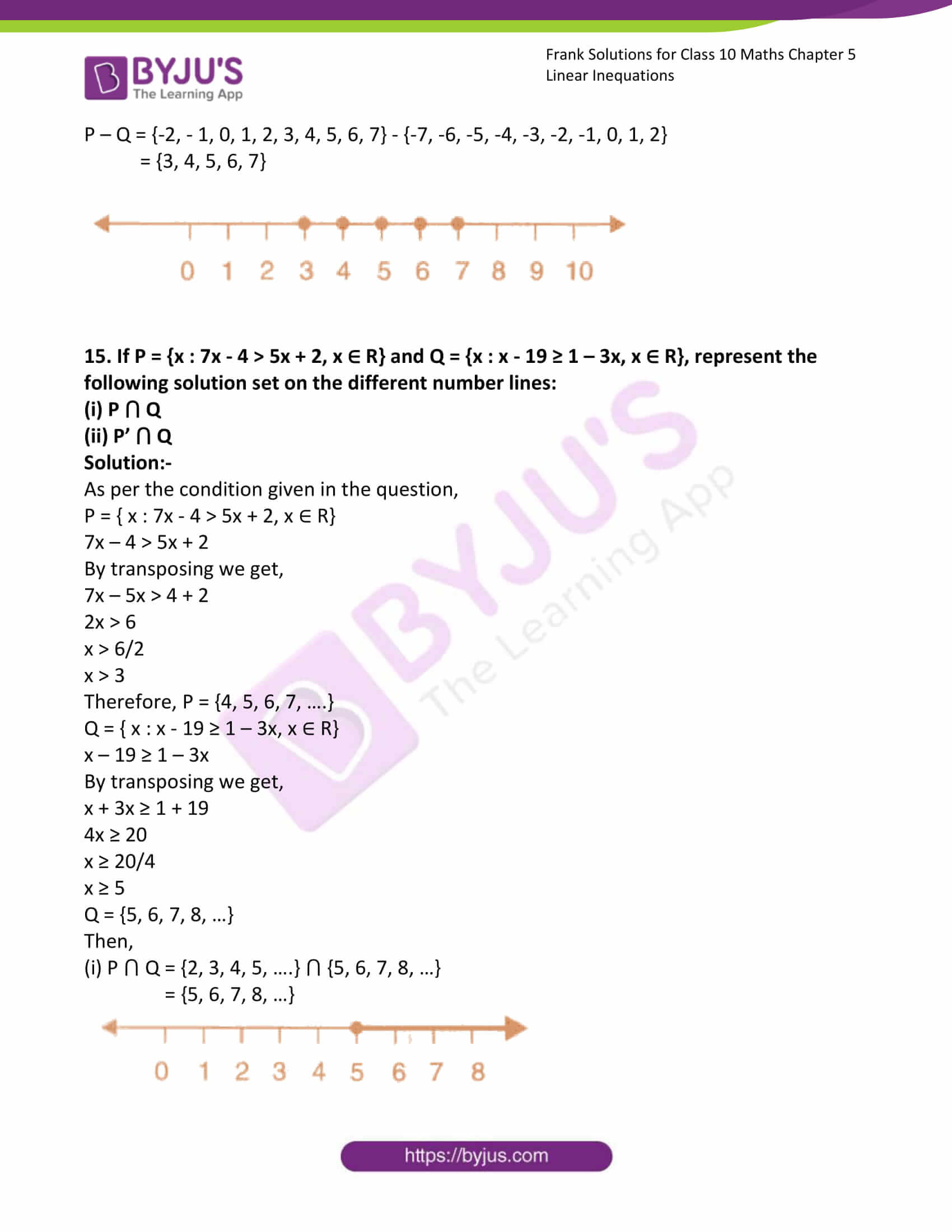### Access answers to Frank Solutions for Class 10 Maths Chapter 5 Linear Inequations

1. Solve for x in the following in equations, if the replacement set is N<10:

(i) x + 5 > 11

Solution:-

x + 5 > 11

By transposing we get,

x > 11 – 5

x > 6

As per the condition given in the question, {x : x ∈ N;N < 10}

Therefore, solution set x = {7, 8, 9}

(ii) 2x + 1 < 17

Solution:-

2x + 1 < 17

By transposing we get,

2x < 17 – 1

x < 16/2

x < 8

As per the condition given in the question, {x : x ∈ N;N < 10}

Therefore, solution set x = {1, 2, 3, 4, 5, 6, 7}

(iii) 3x – 5 ≤ 7

Solution:-

3x – 5 ≤ 7

By transposing we get,

3x ≤ 7 + 5

x ≤ 12/3

x ≤ 4

As per the condition given in the question, {x : x ∈ N;N < 10}

Therefore, solution set x = {1, 2, 3, 4}

(iv) 8 – 3x ≥ 2

Solution:-

8 – 3x ≥ 2

By transposing we get,

3x ≥ 8 – 2

3x ≥ 6

x ≥ 6/3

x ≥ 2

As per the condition given in the question, {x : x ∈ N;N < 10}

Therefore, solution set x = {1, 2}

(v) 5 – 2x < 11

Solution:-

5 – 2x < 11

By transposing we get,

2x > 5 – 11

2x > -6

x > -6/2

x > -3

As per the condition given in the question, {x : x ∈ N;N < 10}

Therefore, solution set x = {1, 2, 3, 4, 5, 6, 7, 8, 9}

2. Solve for x in the following in-equations, if the replacement set is R;

(i) 3x > 12

Solution:-

3x > 12

By cross multiplication we get,

x > 12/3

x > 4

As per the condition given in the question, the replacement set is R.

Therefore, solution set x = {x : x ∈ R; x > 4}

(ii) 2x – 3 > 7

Solution:-

2x – 3 > 7

By transposing we get,

2x > 7 + 3

2x > 10

x > 10/2

x > 5

As per the condition given in the question, the replacement set is R.

Therefore, solution set x = {x : x ∈ R; x > 5}

(iii) 3x + 2 ≤ 11

Solution:-

3x + 2 ≤ 11

By transposing we get,

3x ≤ 11 – 2

3x ≤ 9

x ≤ 9/3

x ≤ 3

As per the condition given in the question, the replacement set is R.

Therefore, solution set x = {x : x ∈ R; x ≤ 3}

(iv) 14 – 3x ≥ 5

Solution:-

14 – 3x ≥ 5

By transposing we get,

3x ≤ 14 – 5

3x ≤ 9

x ≤ 9/3

x ≤ 3

As per the condition given in the question, the replacement set is R.

Therefore, solution set x = {x : x ∈ R; x ≤ 3}

(v) 7x + 11 > 16 – 3x

Solution:-

7x + 11 > 16 – 3x

By transposing we get,

7x + 3x > 16 – 11

10x > 5

x > 5/10

x > ½

x > 0.5

As per the condition given in the question, the replacement set is R.

Therefore, solution set x = {x : x ∈ R; x > 0.5}

(vi) 3x + 25 > 8x – 10

Solution:-

3x + 25 > 8x – 10

By transposing we get,

8x – 3x < 25 + 10

5x < 35

x < 35/5

x < 7

As per the condition given in the question, the replacement set is R.

Therefore, solution set x = {x : x ∈ R; x < 7}

(vii) 2(3x – 5) ≤ 8

Solution:-

2(3x – 5) ≤ 8

6x – 10 ≤ 8

By transposing we get,

6x ≤ 8 + 10

6x ≤ 18

x ≤ 18/6

x ≤ 3

As per the condition given in the question, the replacement set is R.

Therefore, solution set x = {x : x ∈ R; x ≤ 3}

(viii) x + 7 ≥ 15 + 3x

Solution:-

x + 7 ≥ 15 + 3x

By transposing we get,

3x – x ≤ 7 – 15

2x ≤ -8

x ≤ -8/2

x ≤ -4

As per the condition given in the question, the replacement set is R.

Therefore, solution set x = {x : x ∈ R; x ≤ -4}

(ix) 2x – 7 ≥ 5x + 8

Solution:-

2x – 7 ≥ 5x + 8

By transposing we get,

5x – 2x ≤ – 8 – 7

3x ≤ – 15

x ≤ – 15/3

x ≤ – 5

As per the condition given in the question, the replacement set is R.

Therefore, solution set x = {x : x ∈ R; x ≤ – 5}

(x) 9 – 4x ≤ 15 – 7x

Solution:-

9 – 4x ≤ 15 – 7x

By transposing we get,

7x – 4x ≤ 15 – 9

3x ≤ 6

x ≤ 6/3

x ≤ 2

As per the condition given in the question, the replacement set is R.

Therefore, solution set x = {x : x ∈ R; x ≤ 2}

3. Solve for x: 6 – 10x < 36, x ∈ {-3, -2, -1, 0, 1, 2}

Solution:-

From the question it is given that,

6 – 10x < 36

So, by transposing we get,

– 10x < 36 – 6

– 10x < 30

10x > -30

x > – 30/10

x > – 3

As per the condition given in the question, x ∈ {-3, -2, -1, 0, 1, 2}.

Therefore, solution set x = {-2, -1, 0, 1, 2}

4. Solve for x: 3 – 2x ≥ x – 12, x ∈ N

Solution:-

From the question it is given that,

3 – 2x ≥ x – 12

So, by transposing we get,

2x + x ≤ 12 + 3

3x ≤ 15

3x ≤ 15

x ≤ 15/3

x ≤ 5

As per the condition given in the question, x ∈ N.

Therefore, solution set x = {1, 2, 3, 4, 5}

5. Solve for x : 5x – 9 ≤ 15 – 7x, x ∈ W.

Solution:-

From the question it is given that,

5x – 9 ≤ 15 – 7x

So, by transposing we get,

5x + 7x ≤ 15 + 9

12x ≤ 24

x ≤ 24/12

x ≤ 2

As per the condition given in the question, x ∈ W.

Therefore, solution set x = {0, 1, 2}

6. Solve for x : 7 + 5x > x – 13, where x is a negative integer.

Solution:-

From the question it is given that,

7 + 5x > x – 13

So, by transposing we get,

5x – x > -13 – 7

4x > – 20

x > -20/4

x > -5

As per the condition given in the question, x is a negative integer.

Therefore, solution set x = {-4, -3, -2, -1}

7. Solve for x : 5x – 14 < 18 – 3x, x ∈ W.

Solution:-

From the question it is given that,

5x – 14 < 18 – 3x

So, by transposing we get,

5x + 3x < 18 +14

8x < 32

x < 32/8

x < 4

As per the condition given in the question, x is x ∈ W.

Therefore, solution set x = {0, 1, 2, 3}

8. Solve for x : 2x + 7 ≥ 5x – 14, where x is a positive prime number.

Solution:-

From the question it is given that,

2x + 7 ≥ 5x – 14

So, by transposing we get,

5x – 2x ≤ 14 + 7

3x ≤ 21

3x ≤ 21

x ≤ 21/3

x ≤ 7

As per the condition given in the question, x is a positive prime number.

Therefore, solution set x = {2, 3, 5, 7}

9. Solve for x : x/4 + 3 ≤ x/3 + 4, where x is a negative odd number.

Solution:-

From the question it is given that,

x/4 + 3 ≤ x/3 + 4

So, by transposing we get,

x/4 – x/3 ≤ 4 – 3

(3x – 4x)/12 ≤ 1

-x ≤ 12

x ≥ -12

As per the condition given in the question, x is a negative odd number.

Therefore, solution set x = {-11, -9, -7, -5, -3, -1}

10. Solve for x : (x + 3)/3 ≤ (x + 8)/ 4, where x is a positive even number.

Solution:-

From the question it is given that,

(x + 3)/3 ≤ (x + 8)/ 4

So, by cross multiplication we get,

4(x + 3) ≤ 3(x + 8)

4x + 12 ≤ 3x + 24

Now, transposing we get

4x – 3x ≤ 24 – 12

x ≤ 12

As per the condition given in the question, x is a positive even number.

Therefore, solution set x = {2, 4, 6, 8, 10, 12}

11. If x + 17 ≤ 4x + 9, find the smallest value of x, when:

(i) x ∈ Z

Solution:-

From the question,

x + 17 ≤ 4x + 9

So, by transposing we get,

4x – x ≥ 17 – 9

3x ≥ 8

x ≥ 8/3

As per the condition given in the question, x ∈ Z.

Therefore, smallest value of x = {3}

(ii) x ∈ R

Solution:-

From the question,

x + 17 ≤ 4x + 9

So, by transposing we get,

4x – x ≥ 17 – 9

3x ≥ 8

x ≥ 8/3

As per the condition given in the question, x ∈ R.

Therefore, smallest value of x = {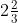}

12. If (2x + 7)/3 ≤ (5x + 1)/4, find the smallest value of x, when:

(i) x ∈ R

Solution:-

From the question,

(2x + 7)/3 ≤ (5x + 1)/4

So, by cross multiplication we get,

4(2x + 7) ≤ 3(5x + 1)

8x + 28 ≤ 15x + 3

Now transposing we get,

15x – 8x ≥ 28 – 3

7x ≥ 25

x ≥ 25/7

As per the condition given in the question, x ∈ R.

Therefore, smallest value of x = {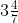}

(ii) x ∈ Z

Solution:-

From the question,

(2x + 7)/3 ≤ (5x + 1)/4

So, by cross multiplication we get,

4(2x + 7) ≤ 3(5x + 1)

8x + 28 ≤ 15x + 3

Now transposing we get,

15x – 8x ≥ 28 – 3

7x ≥ 25

x ≥ 25/7

As per the condition given in the question, x ∈ Z.

Therefore, smallest value of x = {7}

13. Solve the following linear in-equations and graph the solution set on a real number line.

(i) 2x – 11 ≤ 7 – 3x, x ∈ N.

Solution:-

2x – 11 ≤ 7 – 3x

By transposing we get,

2x + 3x ≤ 7 + 11

5x ≤ 18

x ≤ 18/5

x ≤ 3.6

As per the condition given in the question, x ∈ N.

Therefore, solution set x = {1, 2, 3}

Set can be represented in number line as,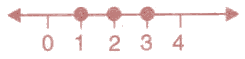(ii) 3(5x + 3) ≥ 2(9x – 17), x ∈ W.

Solution:-

From the question it is given that,

3(5x + 3) ≥ 2(9x – 17)

15x + 9 ≥ 18x – 34

So, by transposing we get,

18x – 15x ≤ 34 + 9

3x ≤ 43

x ≤ 43/3

As per the condition given in the question, x ∈ W.

Therefore, solution set x ≤ 43/3

Set can be represented in number line as,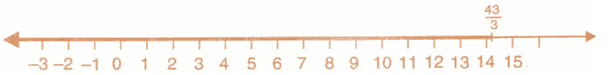(iii) 2(3x – 5) > 5(13 – 2x), x ∈ W.

Solution:-

From the question it is given that,

2(3x – 5) > 5(13 – 2x)

6x – 10 > 65 – 10x

So, by transposing we get,

6x + 10x > 65 + 10

16x > 75

x > 75/16

x >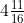As per the condition given in the question, x ∈ W.

Therefore, solution set x >Set can be represented in number line as,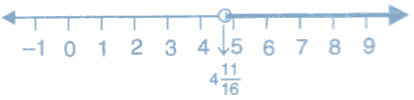(iv) 3x – 9 ≤ 4x – 7 < 2x + 5, x ∈ R.

Solution:-

From the question,

Consider 3x – 9 ≤ 4x – 7

So, by transposing we get,

4x – 3x ≥ -9 + 7

x ≥ -2

Now, consider 4x – 7 < 2x + 5

By transposing we get,

4x – 2x < 5 + 7

2x < 12

x < 12/2

x < 6

As per the condition given in the question, x ∈ R.

Therefore, solution set = [-2 ≤ x < 6]

Set can be represented in number line as,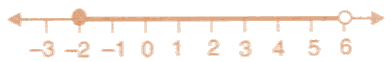(v) 2x – 7 < 5x + 2 ≤ 3x + 14, x ∈ R.

Solution:-

From the question,

Consider 2x – 7 < 5x + 2

By transposing we get,

5x – 2x > – 7 – 2

3x < – 9

x < -9/3

x < -3

Now, consider 5x + 2 ≤ 3x + 14

So, by transposing we get,

5x – 3x ≤ 14 – 2

2x ≤ 12

x ≤ 12/2

x ≤ 6

As per the condition given in the question, x ∈ R.

Therefore, solution set = [-3 < x ≤ 6]

Set can be represented in number line as,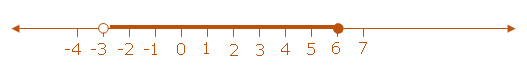(vi) – 3 ≤ ½ – (2x/3) ≤x ∈ N.

Solution:-

From the question,

Consider – 3 ≤ ½ – (2x/3)

-3 ≤ (3 – 4x)/6

– 18 ≤ (3 – 4x)

So, by transposing we get,

– 18 – 3 ≤ – 4x

– 21 ≤ – 4x

x ≤ 21/4

x ≤ 5¼

Now, consider ½ – (2x/3) ≤(3 – 4x)/6 ≤ 8/3

By cross multiplication we get,

3 (3 – 4x) ≤ 48

9 – 12x ≤ 48

By transposing we get,

– 12x ≤ 48 – 9

– 12x ≤ 39

12x ≥ – 39

x ≥ – 39/12

x ≥ -3¼

As per the condition given in the question, x ∈ N.

Therefore, solution set = [-3¼ ≤ x ≤ 5¼]

Set can be represented in number line as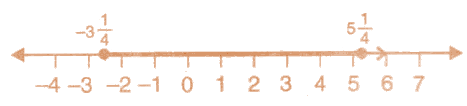(vii) 4¾ ≥ x + 5/6 > 1/3, x ∈ R

Solution:-

From the question,

Consider, 4¾ ≥ x + 5/6

19/4 ≥ (6x + 5)/6

114 ≥ 24x + 20

By transposing we get,

114 – 20 ≥ 24x

94 ≥ 24x

x ≤ 94/24

x ≤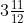Now, consider x + 5/6 > 1/3

(6x + 5)/6 > 1/3

18x + 15 > 6

By transposing we get,

18x > 6 – 15

18x > – 9

x > – 9/18

x > -½

As per the condition given in the question, x ∈ R.

Therefore, solution set = [- ½ < x ≤]

Set can be represented in number line as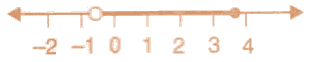(viii) 1/3 (2x – 1) < ¼ (x + 5) < 1/6 (3x + 4), x ∈ R.

Solution:-

From the question it is given that,

Consider 1/3 (2x – 1) < ¼ (x + 5)

By cross multiplication we get,

4(2x – 1) < 3(x + 5)

8x – 4 < 3x + 15

By transposing we get,

8x – 3x < 15 + 4

5x < 19

x < 19/5

x <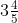Then, consider ¼ (x + 5) < 1/6 (3x + 4)

6(x + 5) < 4(3x + 4)

6x + 30 < 12x + 16

By transposing we get,

6x – 12x < 16 – 30

– 6x < – 14

x >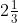As per the condition given in the question, x ∈ R.

Therefore, solution set = [< x <]

Set can be represented in number line as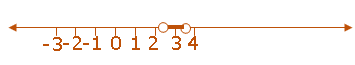(ix) 1/3(5x – 8) ≥ ½ (4x – 7), x ∈ R.

Solution:-

From the question it is given that,

1/3(5x – 8) ≥ ½ (4x – 7)

By cross multiplication we get,

2(5x – 8) ≥ 3(4x – 7)

10x – 16 ≥ 12x – 21

Transposing we get,

12x – 10x ≤ 21 – 16

2x ≤ 5

x ≤ 5/2

x ≤ 2½

As per the condition given in the question, x ∈ R.

Therefore, solution set = {x < – 8}

Set can be represented in number line as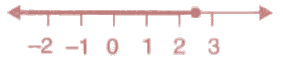(x) 5/4x > 1 + 1/3 (4x – 1), x ∈ R.

Solution:-

From the question,

Consider, (5/4)x > 1 + 1/3 (4x – 1)

(5/4)x > (3 +(4x – 1)/3)

15x > 12 + 16x – 4

By transposing we get,

15x – 16x > 8

– x > 8

x < – 8

As per the condition given in the question, x ∈ R.

Therefore, solution set = {x < – 8}

Set can be represented in number line as,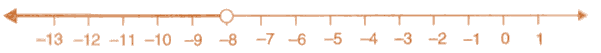14. If P = {x : -3 < x ≤ 7, x ∈ R} and Q = {x : – 7 ≤ x < 3, x ∈ R}, represent the following solution set on the different number lines:

(i) P ⋂ Q

(ii) Q’ ⋂ P

(iii) P – Q

Solution:-

As per the condition given in the question,

P = {x : -3 < x ≤ 7, x ∈ R}

So, P = {-2, – 1, 0, 1, 2, 3, 4, 5, 6, 7}

Then, Q = {x : – 7 ≤ x < 3, x ∈ R}

Q = {-7, -6, -5, -4, -3, -2, -1, 0, 1, 2}

(i) P ⋂ Q = {-2, – 1, 0, 1, 2, 3, 4, 5, 6, 7} ⋂ {-7, -6, -5, -4, -3, -2, -1, 0, 1, 2}

= {-2, -1, 0, 1, 2}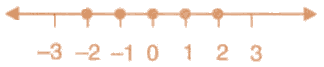(ii) Q’ ⋂ P

Q’ = {3, 4, 5, 6, 7}

Q’ ⋂ P = {3, 4, 5, 6, 7} ⋂ {-2, – 1, 0, 1, 2, 3, 4, 5, 6, 7}

= {3, 4, 5, 6, 7}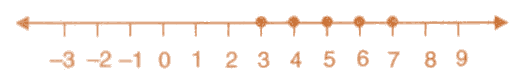(iii) P – Q

P – Q = {-2, – 1, 0, 1, 2, 3, 4, 5, 6, 7} – {-7, -6, -5, -4, -3, -2, -1, 0, 1, 2}

= {3, 4, 5, 6, 7}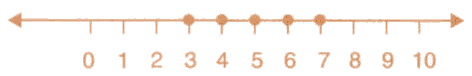15. If P = {x : 7x – 4 > 5x + 2, x ∈ R} and Q = {x : x – 19 ≥ 1 – 3x, x ∈ R}, represent the following solution set on the different number lines:

(i) P ⋂ Q

(ii) P’ ⋂ Q

Solution:-

As per the condition given in the question,

P = { x : 7x – 4 > 5x + 2, x ∈ R}

7x – 4 > 5x + 2

By transposing we get,

7x – 5x > 4 + 2

2x > 6

x > 6/2

x > 3

Therefore, P = {4, 5, 6, 7, ….}

Q = { x : x – 19 ≥ 1 – 3x, x ∈ R}

x – 19 ≥ 1 – 3x

By transposing we get,

x + 3x ≥ 1 + 19

4x ≥ 20

x ≥ 20/4

x ≥ 5

Q = {5, 6, 7, 8, …}

Then,

(i) P ⋂ Q = {2, 3, 4, 5, ….} ⋂ {5, 6, 7, 8, …}

= {5, 6, 7, 8, …}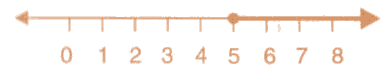(ii) P’ ⋂ Q = {∅}